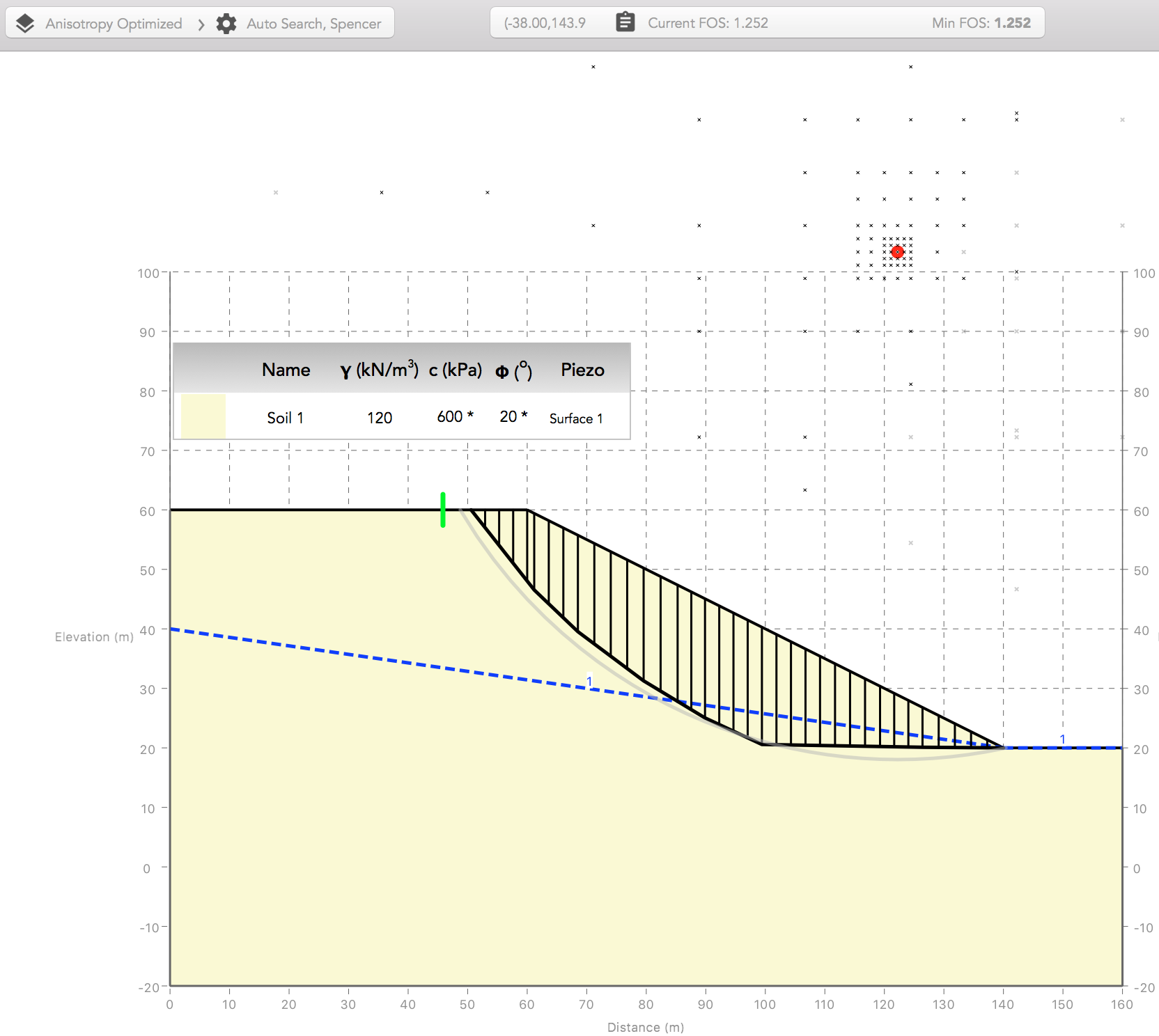• Index
•
•

## Slip Surface Optimization and Anisotropy

In this verification example two slope geometries reviewed by Greco (1996) will be analyzed, to demonstrate the use of the optimization technique and the random optimization search type employed in MobiSlope. The influence of anisotropy in both the slope geometry and the material properties on the critical slip surface shape will be observed.

### Example 1 - Layered Slope

This geometry was originally reviewed by Arai and Tagyo (1985) and has been studied by various authors. It consists of a weaker inclined layer "sandwiched" in between two stronger inclined layers. Greco (1996) obtained a factor of safety of 0.388 with Janbu's Simplified method, using the optimization and slip surface generation methods proposed by the author, which form the basis of the MobiSlope optimization implementation.

First the critical circular slip surface is obtained using the Auto Search. The factor of safety is calculated as 0.426 and the slip surface is shown below.Because the slip surfaces used in the auto search are circular, they do not provide a suitable means to analyze the anisotropy in this geometry. To probe the possibility of a non-circular slip surface with a lower factor of safety, we run a random optimization search, with 10 initial trials. Each trial begins with 4 vertices and will be optimized until it reaches 13 vertices. The minimum factor of safety is calculated as 0.388 and the critical slip surface is shown below. The result is identical to that obtained by Greco (1996).Note that, unlike the circular slip surface, this slip surface passes entirely through the weaker middle layer, the upper part of the surface assuming a convex shape, and the lower part of the surface sliding along the top of the stronger lower material contact. The convex part of the slip surface is achieved by setting the Max Convex Angle parameter to allow convex angles up to 10 degrees. In this case, due to the geometry anisotropy, a slightly convex slip surface shape is kinematically feasible.

### Example 2 - Uniform Slope

This geometry was originally reviewed by Fredlund and Krahn (1977) and has been studied by various authors. In this comparison the slope is considered with a uniform soil profile and a piezometric surface as in case 5. Fredlund and Krahn (1977) obtained a factor of safety of 1.830 with Spencer's method and a (non-critical) circular slip surface. Greco (1996) obtained a factor of safety of 1.744 - 1.751 with Spencer's method, using the optimization and slip surface generation methods proposed by the author, which form the basis of the MobiSlope optimization implementation.

First the critical circular slip surface is obtained using the Auto Search. The factor of safety is calculated as 1.801 and the slip surface is shown below.The critical non-circular slip surface is now analyzed using a random optimization search with 10 initial trials. Each trial begins with 4 vertices and will be optimized until it reaches 13 vertices. The minimum factor of safety is calculated as 1.777 and the critical slip surface is shown below. The result is comparable to that calculated by the author (1.744 - 1751), and is slightly lower than that obtained by the circular slip surface.### Example 3 - Uniform Slope with Material Anisotropy

In order to demonstrate the use of material anisotropy and optimization together, we consider the same case as in example 2, but now we define an Anisotropic Range to model a weaker horizontal bedding plane in the uniform soil. The weaker anisotropic range is defined from β = -1 deg to β = 1 deg, with a cohesion c = 0 kPa and a friction angle ϕ = 20 deg. The soil retains its default (cross-bedded) strength parameters for all other slice base angles.

First the critical circular slip surface is obtained using the Auto Search. The factor of safety is calculated as 1.404 and the slip surface is shown below.The critical circular slip surface is now optimized, beginning with 4 vertices until it reaches 13 vertices. The minimum factor of safety is calculated as 1.252 and the critical slip surface is shown below.Note that the reduction in the factor of safety and the change in shape between the circular and optimized non-circular slip surfaces is now more pronounced after introducing the horizontal anisotropy. Although the circular slip surface contains a horizontal portion and has reduced its factor of safety from 1.801 to 1.404, the non-circular slip surface locates the weak horizontal anisotropy and mutates into a more critical active wedge and horizontal sliding portion, giving a reduction in factor of safety from 1.777 to 1.252.

Download the MobiSlope files with the analysis results for this example here and here.

### References

• Greco, V. R. 1996 . “Efficient Monte Carlo technique for locating critical slip surface.” J. Geotech. Engrg., 122 7 , 517–525.